Praxis Mathematics: Triangles Chapter Exam

Exam Instructions:

Choose your answers to the questions and click 'Next' to see the next set of questions. You can skip questions if you would like and come back to them later with the yellow "Go To First Skipped Question" button. When you have completed the practice exam, a green submit button will appear. Click it to see your results. Good luck!

Answered 0 of 30

Page 1

Question 1 1. In the pictured triangle, identify the altitude(s).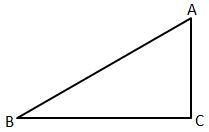Page 2

Question 7 7. The isosceles triangle pictured below shows one angle measuring 40 degrees. What is the measure of angle x?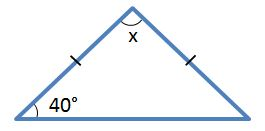Question 9 9. What type of triangle is pictured below?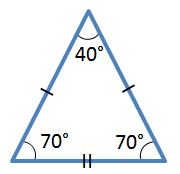Page 3

Question 14 14. In the pictured triangle, which of the following lines is an altitude?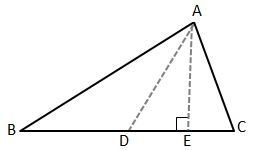Question 15 15. Study the given diagram. Find the value of x, the distance between T and E.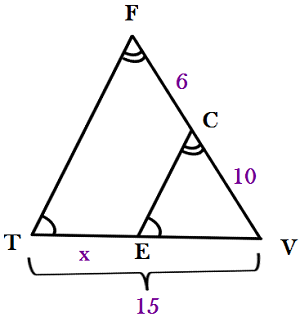Page 4

Question 17 17. Josh claims that these triangles are similar by SSS. Do you agree or disagree with Josh?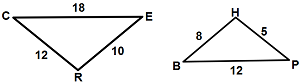Question 20 20. Jerry wants to buy a TV online, but the ad for the TV he wants does not say what the screen size is. He knows that TV screens are measured diagonally. The ad states the height and width of the screen, so he uses the Pythagorean Theorem to determine what the screen size is. What is the screen size of the TV that Jerry wants to buy?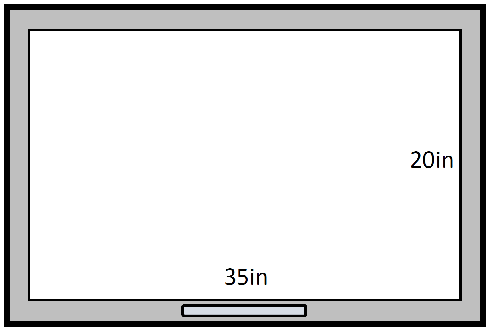Page 5

Question 21 21. Determine if triangle VEZ is similar to triangle MAG.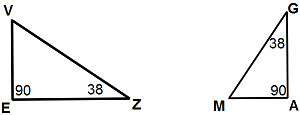Question 22 22. What type of triangle is pictured?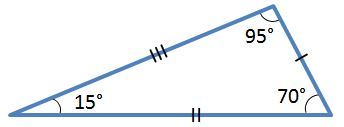Question 24 24. In the right triangle pictured below, what is the value of angle x?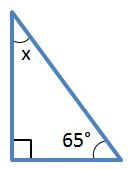Question 25 25. Conclude whether triangle BEN is similar to triangle MAT.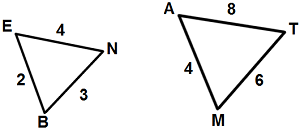Page 6

Question 26 26. The triangle below has an altitude of 10. The longer split of the hypotenuse is 12. What is the measurement of the shorter split of the hypotenuse?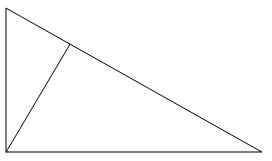Question 28 28. Mary claims that the triangles below are similar by AA. Do you agree or disagree with Mary?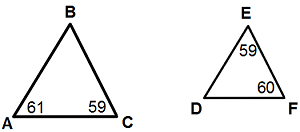Question 29 29. In the picture shown below, there are three lines marking each side of the triangle. These lines give the indication that the triangle is: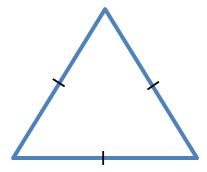Praxis Mathematics: Triangles Chapter Exam Instructions

Choose your answers to the questions and click 'Next' to see the next set of questions. You can skip questions if you would like and come back to them later with the yellow "Go To First Skipped Question" button. When you have completed the practice exam, a green submit button will appear. Click it to see your results. Good luck!

Support